# sklearn.svm.LinearSVC¶

class sklearn.svm.LinearSVC(penalty='l2', loss='squared_hinge', dual=True, tol=0.0001, C=1.0, multi_class='ovr', fit_intercept=True, intercept_scaling=1, class_weight=None, verbose=0, random_state=None, max_iter=1000)[source]

Linear Support Vector Classification.

Similar to SVC with parameter kernel=’linear’, but implemented in terms of liblinear rather than libsvm, so it has more flexibility in the choice of penalties and loss functions and should scale better to large numbers of samples.

This class supports both dense and sparse input and the multiclass support is handled according to a one-vs-the-rest scheme.

Read more in the User Guide.

Parameters: penalty : string, ‘l1’ or ‘l2’ (default=’l2’) Specifies the norm used in the penalization. The ‘l2’ penalty is the standard used in SVC. The ‘l1’ leads to coef_ vectors that are sparse. loss : string, ‘hinge’ or ‘squared_hinge’ (default=’squared_hinge’) Specifies the loss function. ‘hinge’ is the standard SVM loss (used e.g. by the SVC class) while ‘squared_hinge’ is the square of the hinge loss. dual : bool, (default=True) Select the algorithm to either solve the dual or primal optimization problem. Prefer dual=False when n_samples > n_features. tol : float, optional (default=1e-4) Tolerance for stopping criteria. C : float, optional (default=1.0) Penalty parameter C of the error term. multi_class : string, ‘ovr’ or ‘crammer_singer’ (default=’ovr’) Determines the multi-class strategy if y contains more than two classes. "ovr" trains n_classes one-vs-rest classifiers, while "crammer_singer" optimizes a joint objective over all classes. While crammer_singer is interesting from a theoretical perspective as it is consistent, it is seldom used in practice as it rarely leads to better accuracy and is more expensive to compute. If "crammer_singer" is chosen, the options loss, penalty and dual will be ignored. fit_intercept : boolean, optional (default=True) Whether to calculate the intercept for this model. If set to false, no intercept will be used in calculations (i.e. data is expected to be already centered). intercept_scaling : float, optional (default=1) When self.fit_intercept is True, instance vector x becomes [x, self.intercept_scaling], i.e. a “synthetic” feature with constant value equals to intercept_scaling is appended to the instance vector. The intercept becomes intercept_scaling * synthetic feature weight Note! the synthetic feature weight is subject to l1/l2 regularization as all other features. To lessen the effect of regularization on synthetic feature weight (and therefore on the intercept) intercept_scaling has to be increased. class_weight : {dict, ‘balanced’}, optional Set the parameter C of class i to class_weight[i]*C for SVC. If not given, all classes are supposed to have weight one. The “balanced” mode uses the values of y to automatically adjust weights inversely proportional to class frequencies in the input data as n_samples / (n_classes * np.bincount(y)) verbose : int, (default=0) Enable verbose output. Note that this setting takes advantage of a per-process runtime setting in liblinear that, if enabled, may not work properly in a multithreaded context. random_state : int, RandomState instance or None, optional (default=None) The seed of the pseudo random number generator to use when shuffling the data for the dual coordinate descent (if dual=True). When dual=False the underlying implementation of LinearSVC is not random and random_state has no effect on the results. If int, random_state is the seed used by the random number generator; If RandomState instance, random_state is the random number generator; If None, the random number generator is the RandomState instance used by np.random. max_iter : int, (default=1000) The maximum number of iterations to be run. coef_ : array, shape = [n_features] if n_classes == 2 else [n_classes, n_features] Weights assigned to the features (coefficients in the primal problem). This is only available in the case of a linear kernel. coef_ is a readonly property derived from raw_coef_ that follows the internal memory layout of liblinear. intercept_ : array, shape =  if n_classes == 2 else [n_classes] Constants in decision function.

See also

SVC
Implementation of Support Vector Machine classifier using libsvm: the kernel can be non-linear but its SMO algorithm does not scale to large number of samples as LinearSVC does. Furthermore SVC multi-class mode is implemented using one vs one scheme while LinearSVC uses one vs the rest. It is possible to implement one vs the rest with SVC by using the sklearn.multiclass.OneVsRestClassifier wrapper. Finally SVC can fit dense data without memory copy if the input is C-contiguous. Sparse data will still incur memory copy though.
sklearn.linear_model.SGDClassifier
SGDClassifier can optimize the same cost function as LinearSVC by adjusting the penalty and loss parameters. In addition it requires less memory, allows incremental (online) learning, and implements various loss functions and regularization regimes.

Notes

The underlying C implementation uses a random number generator to select features when fitting the model. It is thus not uncommon to have slightly different results for the same input data. If that happens, try with a smaller tol parameter.

The underlying implementation, liblinear, uses a sparse internal representation for the data that will incur a memory copy.

Predict output may not match that of standalone liblinear in certain cases. See differences from liblinear in the narrative documentation.

References

LIBLINEAR: A Library for Large Linear Classification

Examples

>>> from sklearn.svm import LinearSVC
>>> from sklearn.datasets import make_classification
>>> X, y = make_classification(n_features=4, random_state=0)
>>> clf = LinearSVC(random_state=0, tol=1e-5)
>>> clf.fit(X, y)
LinearSVC(C=1.0, class_weight=None, dual=True, fit_intercept=True,
intercept_scaling=1, loss='squared_hinge', max_iter=1000,
multi_class='ovr', penalty='l2', random_state=0, tol=1e-05, verbose=0)
>>> print(clf.coef_)
[[0.085... 0.394... 0.498... 0.375...]]
>>> print(clf.intercept_)
[0.284...]
>>> print(clf.predict([[0, 0, 0, 0]]))



Methods

 decision_function(X) Predict confidence scores for samples. densify() Convert coefficient matrix to dense array format. fit(X, y[, sample_weight]) Fit the model according to the given training data. get_params([deep]) Get parameters for this estimator. predict(X) Predict class labels for samples in X. score(X, y[, sample_weight]) Returns the mean accuracy on the given test data and labels. set_params(**params) Set the parameters of this estimator. sparsify() Convert coefficient matrix to sparse format.
__init__(penalty='l2', loss='squared_hinge', dual=True, tol=0.0001, C=1.0, multi_class='ovr', fit_intercept=True, intercept_scaling=1, class_weight=None, verbose=0, random_state=None, max_iter=1000)[source]
decision_function(X)[source]

Predict confidence scores for samples.

The confidence score for a sample is the signed distance of that sample to the hyperplane.

Parameters: X : array_like or sparse matrix, shape (n_samples, n_features) Samples. array, shape=(n_samples,) if n_classes == 2 else (n_samples, n_classes) Confidence scores per (sample, class) combination. In the binary case, confidence score for self.classes_ where >0 means this class would be predicted.
densify()[source]

Convert coefficient matrix to dense array format.

Converts the coef_ member (back) to a numpy.ndarray. This is the default format of coef_ and is required for fitting, so calling this method is only required on models that have previously been sparsified; otherwise, it is a no-op.

Returns: self : estimator
fit(X, y, sample_weight=None)[source]

Fit the model according to the given training data.

Parameters: X : {array-like, sparse matrix}, shape = [n_samples, n_features] Training vector, where n_samples in the number of samples and n_features is the number of features. y : array-like, shape = [n_samples] Target vector relative to X sample_weight : array-like, shape = [n_samples], optional Array of weights that are assigned to individual samples. If not provided, then each sample is given unit weight. self : object
get_params(deep=True)[source]

Get parameters for this estimator.

Parameters: deep : boolean, optional If True, will return the parameters for this estimator and contained subobjects that are estimators. params : mapping of string to any Parameter names mapped to their values.
predict(X)[source]

Predict class labels for samples in X.

Parameters: X : array_like or sparse matrix, shape (n_samples, n_features) Samples. C : array, shape [n_samples] Predicted class label per sample.
score(X, y, sample_weight=None)[source]

Returns the mean accuracy on the given test data and labels.

In multi-label classification, this is the subset accuracy which is a harsh metric since you require for each sample that each label set be correctly predicted.

Parameters: X : array-like, shape = (n_samples, n_features) Test samples. y : array-like, shape = (n_samples) or (n_samples, n_outputs) True labels for X. sample_weight : array-like, shape = [n_samples], optional Sample weights. score : float Mean accuracy of self.predict(X) wrt. y.
set_params(**params)[source]

Set the parameters of this estimator.

The method works on simple estimators as well as on nested objects (such as pipelines). The latter have parameters of the form <component>__<parameter> so that it’s possible to update each component of a nested object.

Returns: self
sparsify()[source]

Convert coefficient matrix to sparse format.

Converts the coef_ member to a scipy.sparse matrix, which for L1-regularized models can be much more memory- and storage-efficient than the usual numpy.ndarray representation.

The intercept_ member is not converted.

Returns: self : estimator

Notes

For non-sparse models, i.e. when there are not many zeros in coef_, this may actually increase memory usage, so use this method with care. A rule of thumb is that the number of zero elements, which can be computed with (coef_ == 0).sum(), must be more than 50% for this to provide significant benefits.

After calling this method, further fitting with the partial_fit method (if any) will not work until you call densify.

## Examples using sklearn.svm.LinearSVC¶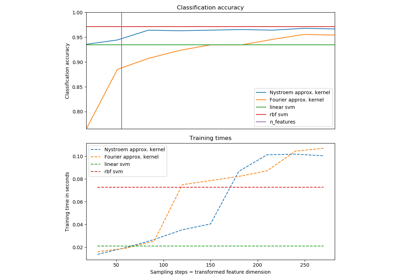Explicit feature map approximation for RBF kernels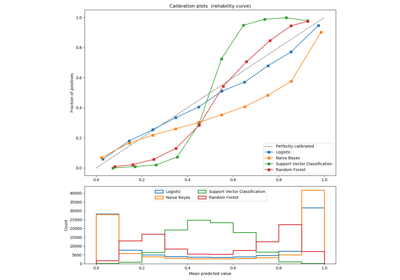Comparison of Calibration of Classifiers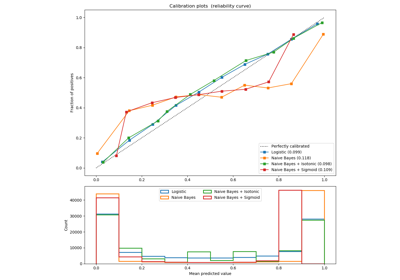Probability Calibration curves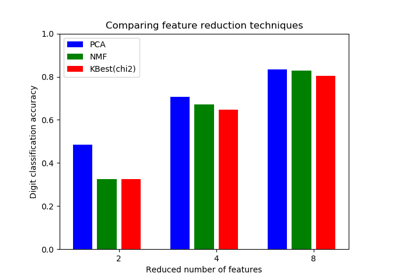Selecting dimensionality reduction with Pipeline and GridSearchCVColumn Transformer with Heterogeneous Data Sources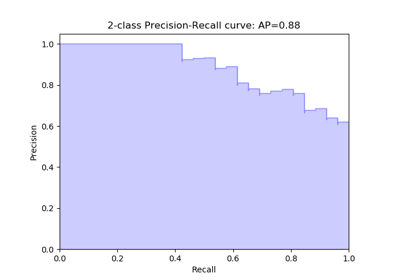Precision-Recall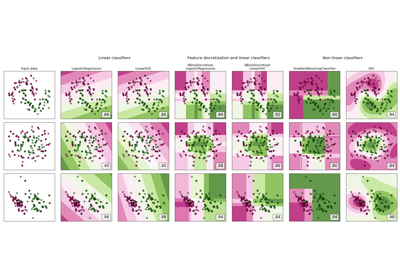Feature discretization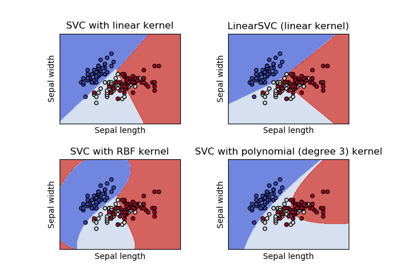Plot different SVM classifiers in the iris dataset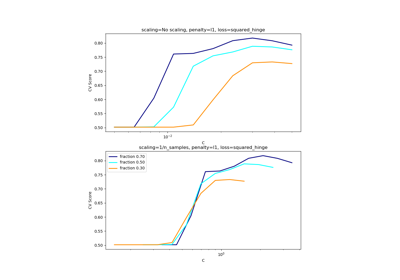Scaling the regularization parameter for SVCs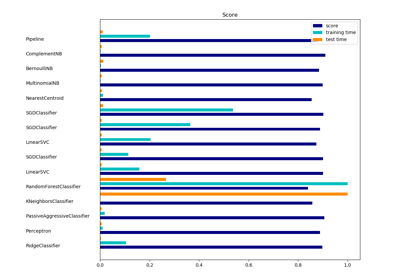Classification of text documents using sparse features